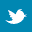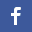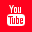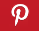Completely Solved C, C++ Programs Assignment.Quick Search Database Videos Tutorials Ebooks Forums FAQ Aboutus Household Industrial Manufacturing Service Shopping Transportation### C Program to calculate LCM, HCF of n numbers

 Filed Under: C Assignments

Problem statement:
Program to calculate LEAST COMMON MULTIPLE and HIGHEST COMMON FACTOR of n numbers. n is taken as input from the user.

Theory:
The H.C.F. of two numbers is the greatest number among all the common factors of two numbers. We calculate the H.C.F. of n number by repeatedly calculating H.C.F. of two numbers, storing it in a variable (say h) and further finding the H.C.F. of h and the next number. The L.C.M. of two numbers is the least possible number divisible by both the number. We calculate the L.C.M. of n number by the application of procedure to calculate L.C.M. of two numbers, just as we applied for finding H.C.F.

Algorithm:
Algorithm hcf(int a,int b)
int lcm(int a,int b,int x)                         /*function to find lcm of a and b*/
if(a%b)
lcm(a+x,b,x);
else
return a;
}
Algorithm lcm(int a,int b,int x)
int hcf(int a,int b)                                 /*function to find hcf of a and b*/
{
if(!a)
return b;
if(!b)
return a;
hcf(b,a%b);
}

PROGRAM LISTING:
/*program to find lcm and hcf of n numbers*/
#include<stdio.h>
#include<conio.h>
int hcf(int a,int b)                              /*function to find hcf of a and b*/
{
if(!a)
return b;
if(!b)
return a;
hcf(b,a%b);
}                                                               /*end of function hcf*/
int lcm(int a,int b,int x) /*function to find lcm of a and b*/
{
if(a%b) lcm(a+x,b,x);
else return a;
}                                                             /*end of function lcm*/
void main()
{
int a,n,i,l,h;
clrscr();
printf("nEnter the number of element :"); /*reading the number of element n*/
scanf("%d",&n);
printf("nEnter the 1 Element:");
scanf("%d",&a);
l=a;
h=a;
printf("n************************************n");
for(i=1;i<n;i++)
printf("nEnter the %d element :",i+1);
scanf("%d",&a[i]);
printf("n************************************n");
if(l<a[i]) l=lcm(a[i],l,a[i]);                             /*function call to find lcm*/
else l=lcm(l,a[i],l);                                          /*function call to find lcm*/
h=hcf(h,a[i]);                                                  /*function call to find hcf*/
}
printf("nLEAST COMMON MULTIPLE = %dn",l);
printf("nHIGHEST COMMON FACTOR = %d",h);
getch();
}                                                                 /*end of main*/

Output:
Enter the number of element :5
Enter the 1 element :5
************************************
Enter the 2 element :10
***********************************
Enter the 3 element :15
************************************
Enter the 4 element :20
************************************
Enter the 5 element :25
************************************
LEAST COMMON MULTIPLE = 300
HIGHEST COMMON FACTOR = 5

Discussion:
• The two function hcf() and lcm() make use of recursion in order to calculate the hcf and lcm of two numbers respectively. We utilize these two functions repeatedly for calculating the required hcf and lcm of n numbers. the use of recursive functions largely reduce the program code.
• The above algorithm can be implemented in different way to reduce the complexity or better performance.Back to main directory:Software Practical

 @import url(http://www.google.com/cse/api/branding.css);